# 1(b) .3 - VisualizationPrinter-friendly version

To understand thousands of rows of data in a limited time there is no alternative to visual representation. Objective of visualization is to reveal the hidden information through simple charts and diagrams. Visual representation of data is the first step towards data exploration and formulation of analytical relationship among the variables. In a whirl of complex and voluminous data, visualization in one, two and three dimension helps data analysts to sift through data in a logical manner and understand the data dynamics. It is instrumental in identifying patterns and relationships among groups of variables. Visualization techniques depend on the type of variables. Techniques available to represent nominal variables are generally not suitable for visualizing continuous variables and vice versa. Data often contains complex information. It is easy to internalize complex information through visual mode. Graphs, charts and other visual representation provide quick and focused summarization.

### Tools for Displaying Single Variables

##### Histogram

Histograms are the most common grapical tool to represent continuous data. On the horizontal axis the range of the sample is plotted. On the vertical axis is plotted the frequencies or relative frequencies of each class. The class width has an impact on the shape of the histogram. The histograms in the previous section were drawn from a random sample generated from theoretical distributions. Here we consider a real example to construct histograms.

The data set used for this purpose is the Wage data that is included in the ISLR package in R. A full description of the data is given in the package. The following R code produces the figure below which illustrates the distribution of wage for all 3000 workers.

Sample R code for Distribution of Wage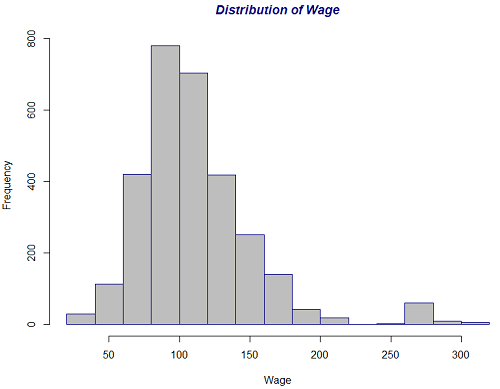The data is mostly symmetrically distributed but there is a small bimodality in the data which is indicated by a small hump towards the right tail of the distribution.

The data set contains a number of categorical variables one of which is Race. A natural question is whether the wage distribution is the same across Race. There are several libraries in R which may be used to construct histograms across levels of a categorical variables and many other sophisticated graphs and charts. One such library is ggplot2. Details of functionalities of this library will be given in the R code below.

Sample R Code for Histogram by Race

In the following figures histograms are drawn for each Race separately.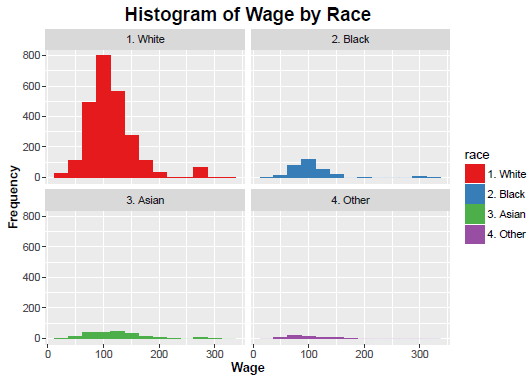Because of huge disparity among the counts of the different races, the above histograms may not be very informative. Code for an alternative visual display of the same information is shown below, followed by the plot.

Sample R Code for Histograms by Race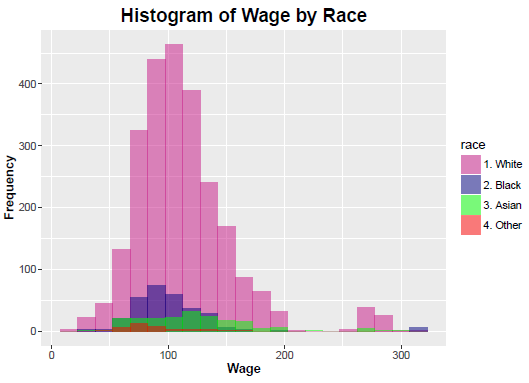The second type of histogram also may not be the best way of presenting all the information. However further clarity is seen in the small concentration at the right tail.

##### Boxplot

Boxplot is used to describe shape of a data distribution and especially to identify outliers. Typically an observation is an outlier if it is either less than Q1 - 1.5 IQR or greater than Q3 + 1.5 IQR, where IQR is the inter-quartile range defined as Q3 - Q1. This rule is conservative and often too many points are identified as outliers. Hence sometimes only those points outside of [Q1 - 3 IQR, Q3 + 3 IQR] are only identified as outliers.

Sample R Code for Boxplot of Distribution of Wage

Here is the boxplot that results: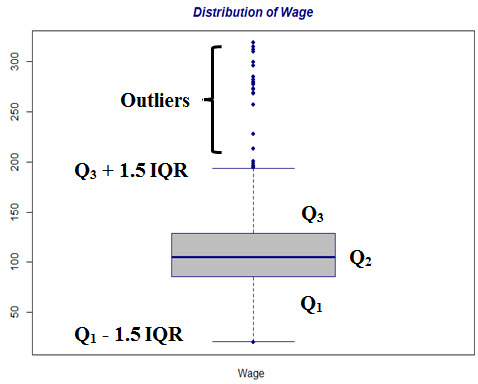The boxplot of the Wage distribution clearly identifies many outliers. It is a reflection of the histogram depicting the distribution of Wage. The story is clearer from the boxplots drawn on the wage distribution for individual races.  Here is the R code:

Sample R Code for Boxplot of Wage by Race

Here is the boxplot that results: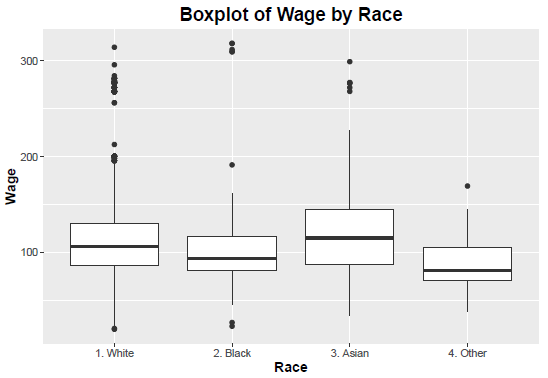### Tools for Displaying Relationships Between Two Variables

#### Scatterplot

The most standard way to visualize relation between two variables is a scatterplot. It shows the direction and strength of association between two variables, but does not quantify. Scatterplots also help to identify unusual observations. In the previous section (Section 1(b).2) a set of scatterplots are drawn for different values of the correlation coefficient. The data there is generated from a theoretical distribution of multivariate normal distribution with various values of the correlation paramater. Below is the R code used to obtain a scatterplot for these data:

Sample R Code for Relationship of Age and Wage

The following is the scatterplot of the variables Age and Wage for the Wage data.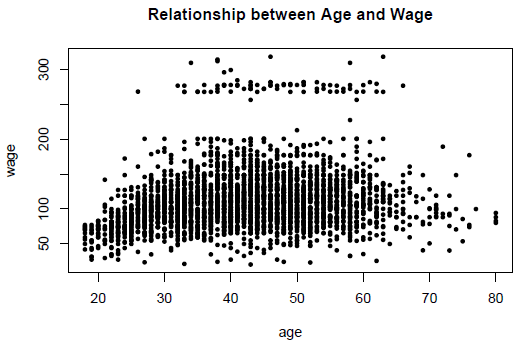It is clear from the scatterplot that the Wage does not seem to depend on Age very strongly. However a set of points is towards top are very different from the rest. A natural follow-up question is whether Race has any impact on the Age-Wage dependency, or the lack of it.  Here is the R code and then the new plot:

Sample R Code for Relationship of Age and Wage by RaceWe have noted before that the disproportionately high number of Whites in the data masks the effects of the other races. There does not seem to be any association between Age and Wage, controlling for Race.

#### Contour plot

This is useful when a continuous attribute is measured on a spatial grid. They partition the plane into regions of similar values. The contour lines that form the boundaries of these regions connect points with equal values.  In spatial statistics contour plots have a lot of applications.

Contour plots join points of equal probability. Within the contour lines concentration of bivariate distribution is the same. One may think of the contour lines as slices of a bivariate density, sliced horizontally. Contour plots are concentric; if they are perfect circles then the random variables are independent. The more oval shaped they are, the farther they are from independence. Note the conceptual similarity in the scatterplot series in Sec 1.(b).2. In the following plot the two disjoint shapes in the interior-most part indicate that a small part of the data is very different from the rest.

Here is the R code for the contour plot that follows:

Sample R Code for Contour Plot of Age and Wage### Tools for Displaying More Than Two Variables

#### Scatterplot Matrix

Displaying more than two variables on a single scatterplot is not possible. Scatterplot matrix is one possible visualization of three or more continuous variables taken two at a time.

The data set used to display scatterplot matrix is the College data that is included in the ISLR package. A full description of the data is given in the package.  Here is the R code for the scatterplot matrix that follows:

Sample R Code for Scatterplot Matrix of College Attributes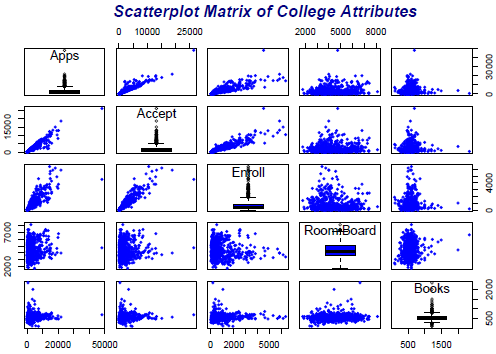#### Parallel Coordinates

An innovative way to present multiple dimensions in the same figure is by using parallel coordinate systems. Each dimension is presented by one coordinate and instead of plotting coordinates at right angle to one another, each coordinate is placed side-by-side. The advantage of such arrangement is that, many different continuous and discrete variables can be handled within parallel coordinate system, but if the number of observations is too large, the profiles do not separate out from one another and patterns may be missed.

The illustration below corresponds to the Auto data from ISLR package. Only 35 cars are considered but all dimensions are taken into account. The cars considered are different varieties of Toyota and Ford, categorized into two groups: produced before 1975 and produced in 1975 or after. The older models are represented by dotted lines whereas the newer cars are represented by dashed lines. The Fords are represented by blue colour and Toyotas are represented by pink color.  Here is the R code for the profile plot of this data that follows:

Sample R Code for Profile Plot of Toyota and Ford Cars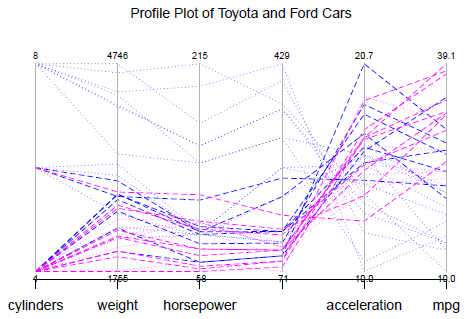The differences among the four groups is very clear from the figure. Early Ford models had 8 cylinders, were heavy and had high horsepower and displacement. Naturally they had low MPG and less time to accelerate. No Toyota belonged to this category. All Toyota cars are built after 1975, have 4 cylinders (one exception only) and on MPG performance belongs to the upper half of the distribution. Note that only 35 cars are compared in the profile plot. Hence each car can be followed over all the attributes. However had the number of observations been higher, the distinction among the profiles would have been lost and the plot would not be informative.

#### Interesting Multivariate Plots

Following are some interesting visualization of multivariate data. In Star Plot, stars are drawn according to rules as defined on the characteristics. Each axis represents one attribute and the solid lines represent each item’s value on that attribute. All attributes of the observations are possible to be represented; however for the sake of clarity on the graph only 10 attributes are chosen.

Again, the starplot follows the R code for generating the plot:

Sample R Code for Starplot of College Data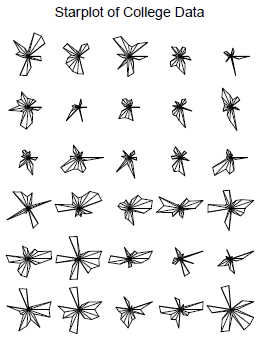Another interesting plot technique with multivariate data is Chernoff Face where attributes of each observation are used to draw different feature of the face. A comparison of 30 colleges and universities from the College dataset is compared below.

Again, R code and then the plot follows:

Sample R Code for Comparison of Colleges and Universities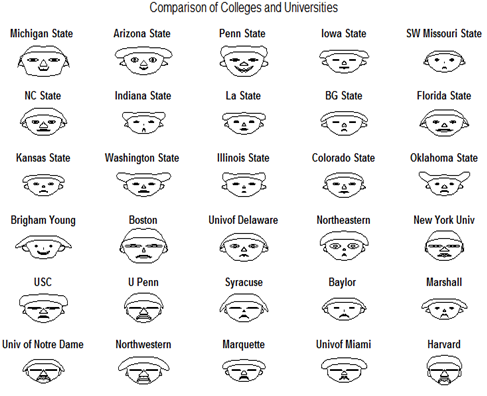For comparison of a small number of observations on up to 15 attributes, Chernoff’s face is a useful technique. However, whether two items are more similar or less, depends on interpretation.